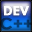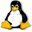Home Latest News Archive Tutorials C++ 2 3 4 Win32 Java OpenGL GLUT OpenGL ES 2 3 4 DirectX VB.NET HTML Photoshop 3dsmax Forums Projects Modelling Links About Us Contact Search Recent Tutorials C / C++ Win32 OpenGL OpenGL ES GLUT## Enumerations

Tutorials > C++ > Enumerations

### Introduction

Enumerations have a similar function to that of constants. They allow you to give a special name to a given integer value. This simplifies your code as you can now create something such as ERROR_CODE_MISSING_FILE instead of using an integer such as 211. You may also find that you are able to code faster as you are able to remember the identifier far quicker than the integer.

### How are Enumerations defined?

An enumeration is created using the following syntax :

enum enumName     { IDENTIFIERS SEPARATED BY COMMAS };

This will assign integer values from 0 to each of the identifiers given. Specific values can also be given by placing a = ? after the identifier where ? is the integer value. This will appear more clearly below.

Contents of main.cpp :

```#include <iostream>
#include <stdlib.h>

using namespace std;

int main()
{
```

We start by creating an enumeration for the days of the week. The code below will give Monday the value of 0 and each successive identifier will increase by 1 resulting in Sunday being equal to 6.

```	enum Weekdays
{
Monday,
Tuesday,
Wednesday,
Thursday,
Friday,
Saturday,
Sunday
};
```

You are now able to use the Weekdays enumeration as a normal data type. We initialize a Weekdays variable with a value of Saturday and print out the value which gives the expected value of 5.

```	Weekdays day = Saturday;
cout << day << endl;
```

You may not want your identifiers to be given values from 0 upwards. The code below shows how you can give specific values to your identifiers. An Angle enumeration is created with identifiers being increased by 90 each time.

```	enum Angle
{
A_UP = 0,
A_RIGHT = 90,
A_DOWN = 180,
A_LEFT = 270
};
```

The code below shows how you can still use your identifier as a normal integer. If you divide the variable angle by 2, the value of 45 is printed out.

```	Angle angle = A_RIGHT;

cout << "half 90 = "
<< angle / 2 << endl;
```

In the next segment of code, it is shown how you are able to typecast a normal integer value to store it in an enumeration variable.

```	angle = (Angle)180;
cout << angle << endl;
```

Our last enumeration shows how you can give some identifiers specific integer values while leaving the rest of the identifiers to determine their own values. Each identifier that is not given a specific value is given the same value as the previous identifier + 1. The value of A4 is therefore given as 0 + 3 = 3.

```	enum ID
{
A1 = 0,
A2,
A3,
A4,
B1 = 10,
B2
};

cout << A4 << endl;

system("pause");

return 0;
}```

You should now be able to create enumerations to simplify your code and make coding both easier and faster. Enumerations are extremely handy in scenarios where error codes or a group of some type of static identifiers are needed.

Please let me know of any comments you may have : Contact Me

 Source Files :< Tutorial 30 - Command Line Arguments Tutorial 32 - Character Strings >

All Rights Reserved, © Zeus Communication, Multimedia & Development 2004-2005

Read the Disclaimer